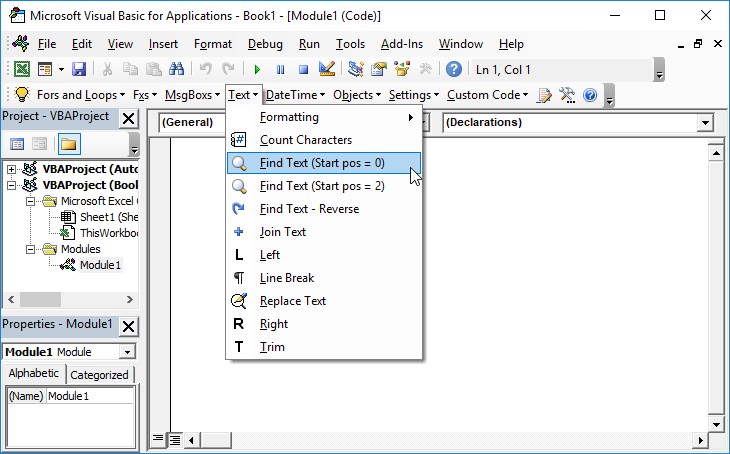# VBA – Extract Number From String

## Extract Numerical Portion of String

The following function will extract the numerical portion from a string:

``````Function Extract_Number_from_Text(Phrase As String) As Double
Dim Length_of_String As Integer
Dim Current_Pos As Integer
Dim Temp As String
Length_of_String = Len(Phrase)
Temp = ""
For Current_Pos = 1 To Length_of_String
If (Mid(Phrase, Current_Pos, 1) = "-") Then
Temp = Temp & Mid(Phrase, Current_Pos, 1)
End If
If (Mid(Phrase, Current_Pos, 1) = ".") Then
Temp = Temp & Mid(Phrase, Current_Pos, 1)
End If
If (IsNumeric(Mid(Phrase, Current_Pos, 1))) = True Then
Temp = Temp & Mid(Phrase, Current_Pos, 1)
End If
Next Current_Pos
If Len(Temp) = 0 Then
Extract_Number_from_Text = 0
Else
Extract_Number_from_Text = CDbl(Temp)
End If
End Function``````

So for example:

Extract_Number_from_Text(“uuigguo 995”) will return 995
Extract_Number_from_Text(“uuigguo 0.12995”) will return 0.12995
Extract_Number_from_Text(“yu00.000456”) will return 0.000456
=Extract_Number_from_Text(“juii-0009.9987iihiii”) will return -0.9987
If there is no number in the phrase then a zero is returned.